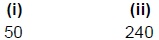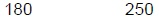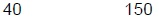# User Forum

Subject :IMO    Class : Class 4

Rakesh made the bar graph for different coloured marbles. By mistake he interchanged the bars for green colour and red colour. Consider the given bar graph and answer the following questions.(i) What is the actual difference between the number of red coloured and yellow coloured marbles?
(ii) What is the sum of brown, green and blue coloured marbles he actually has?

ABCDClass : Class 1

(C):

After interchanging the bars for green
colour and red colour,
Number of brown colour marbles = 50
Number of green colour marbles = 20
Number of blue colour marbles = 80
Number of red colour marbles = 110
Number of yellow colour marbles = 60
(i) Actual difference between the number of red coloured and yellow coloured marbles = 110 - 60 = 50
(ii) Sum of brown, green and blue coloured marbles he actually has = 50 + 20 + 80 = 150

## Ans 2:

Class : Class 4
As per given graph Red colour value is 20 and green colour value is 110

Class : Class 7

## Ans 4:

Class : Class 6
it is written consider the given bar graph